# Basics Of Standard Fractions

A fraction represents a part of a whole or, more generally, any number of equal parts. In this quiz, different questions related to some standard fractions like 1/2, 1/3 are asked to clear the basic concept of fraction to any student. This quiz includes various multiple choice questions are added in the quiz including various pictures related to the question to let students understand the concept of fractions comfortably.

Start Quiz

Select all the figures that show equal parts.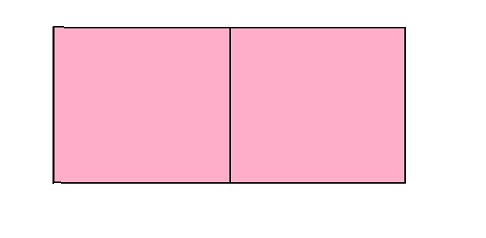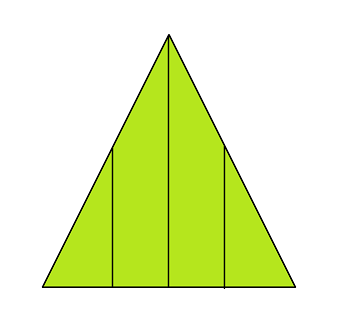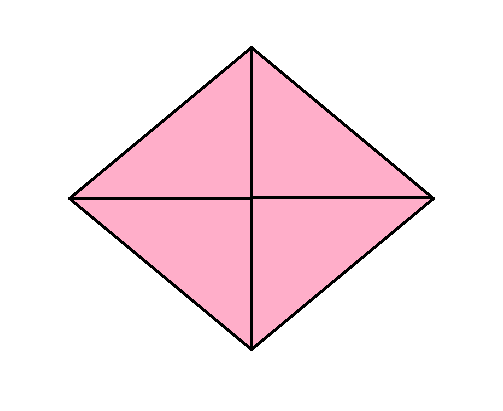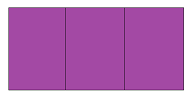Which figure shows 1/3rd fraction?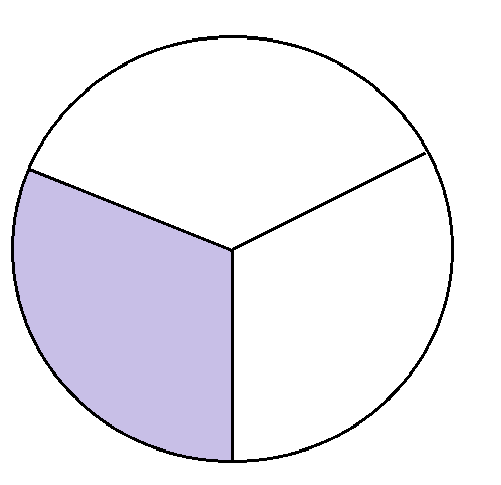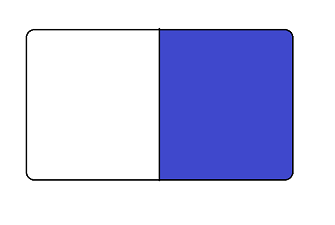Which figure shows 1/2th fraction?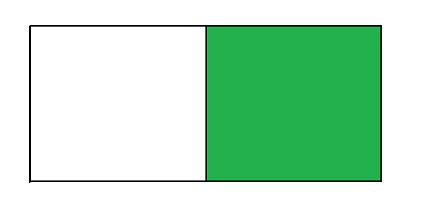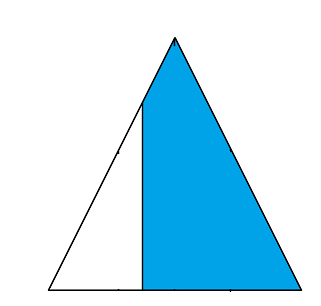Which figure shows 1/2th fraction?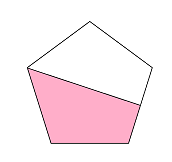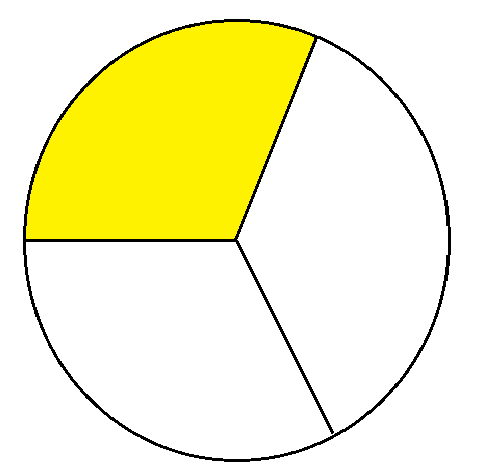Which figure shows 1/3rd fraction?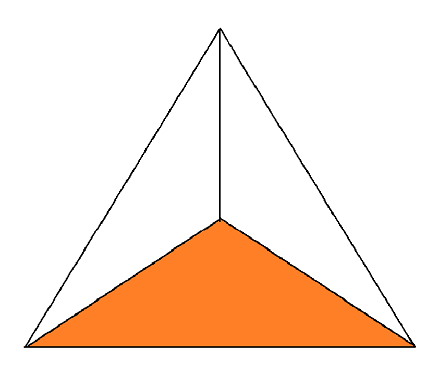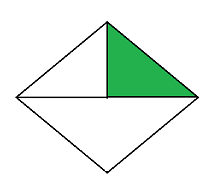Which figure shows 1/4th fraction?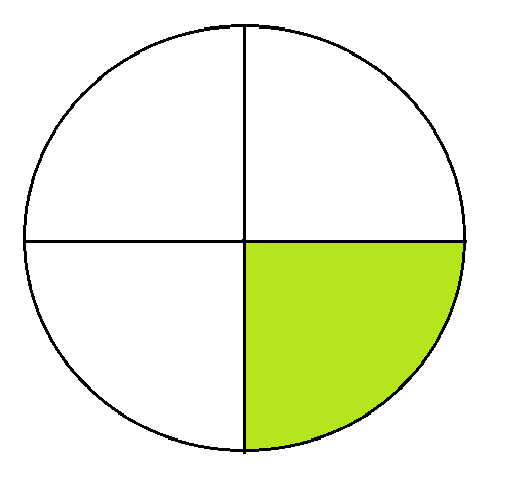Which figure shows 1/3rd fraction?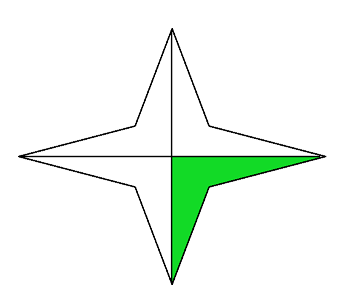What is the fraction of the colored part?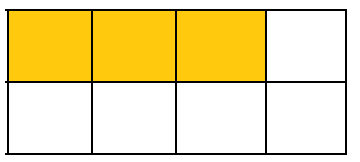4/5

3/8

2/7

1/8

What is the fraction of the colored part?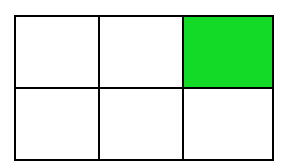1/5

1/4

1/6

1/8

Select all the figures that show equal parts.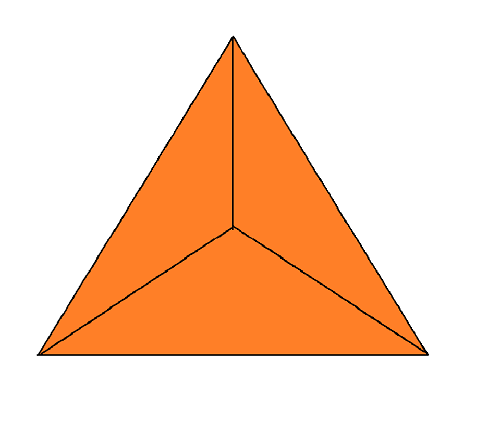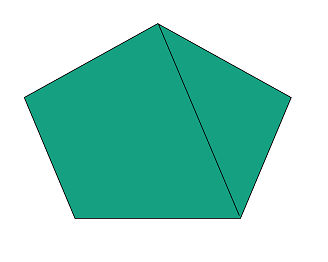Quiz/Test Summary
Title: Basics Of Standard Fractions
Questions: 10
Contributed by: